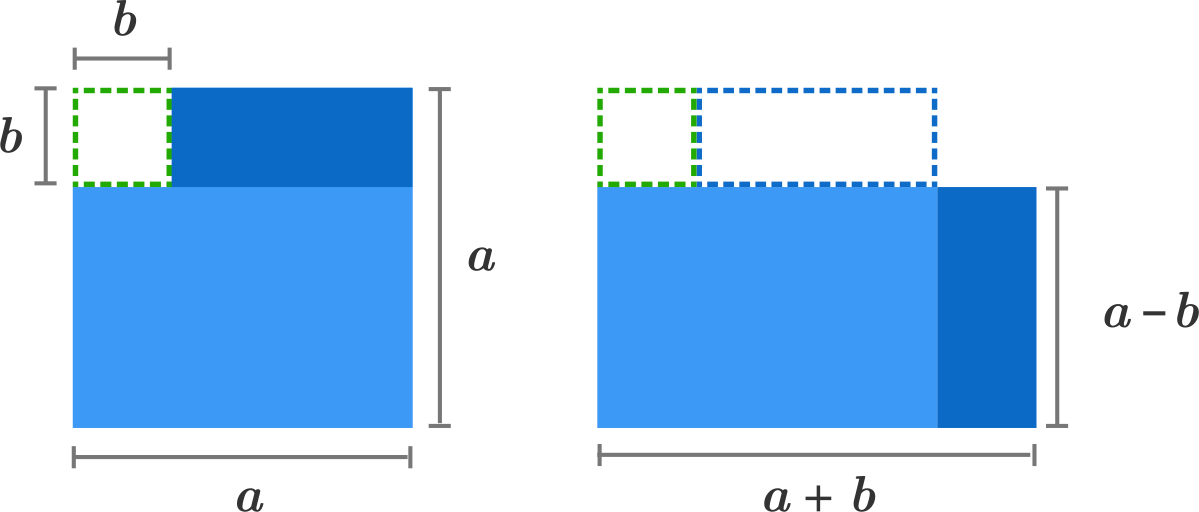# Problems of the Week

Contribute a problem

# 2017-02-06 Basic

There is a math teacher in a school whose favorite number is a positive integer (let's call it $x).$ She told her 5 students--Alice, Beatrice, Candice, Denise, Eunice--the number $x,$ and the following conversation took place:

Alice: "$x+1$ is not divisible by 5."
Beatrice: "$x+2$ is not divisible by 5."
Candice: "$x+3$ is not divisible by 5."
Denise: "$x+4$ is not divisible by 5."
Eunice: "$x+5$ is not divisible by 5."

Without knowing $x$, can we guarantee that at least one of these 5 students is lying?What is the surface area of a cube with a 10-meter space diagonal?James arrived home after a party and realized that he had forgot to turn off the knob of the gas line. There is a strong smell of liquefied petroleum gas in his apartment.

What should he do to avoid any accidents?

1. Turn off the knob of the gas line.
2. Open all the windows.
3. Turn on the exhaust fan.
4. Turn off all the electrical appliances.

Evaluate $\frac{ 63^4 - 37^4 } { 63^2 + 37^2 }.$

Hint: Use the difference of two squares identity.A car is driving at $100\text{ mph}$ down a straight road. What is the speed at the point on the tire where the wheel touches the ground?Note: Assume that the tires are not slipping or skidding.

×